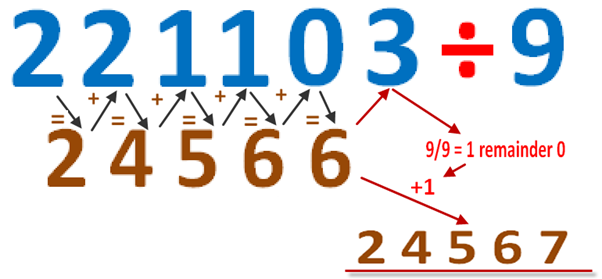Division by 9

This is a cool trick on how to easily divide by 9.

Let us use 5211 for example

1. The 1st digit of the answer will be the first (left most) digit of the number being divided .  5
Final answer so far : 5

2. The next digit will be this answer (5) digit plus the next digit of the number being divided.  5+2=7
Final answer so far :  57

3. The third digit is the previous answer (7) plus the next digit of the number being divided: 7+1 = 8
Final answer so far :  578

4.  Now is the last step as one digit remains for the number being divided.  Like before add it to the previous answer
8+1=9.  But we do not write down 9, but instead we see what remainder it gives from 9 and add it to our final answer.  Since 9 goes 1 times in 9,we add this1  to our answer.
Final answer  :  578+1= 579

The picture bellow shows an illustration when 221103 is divided by 9